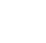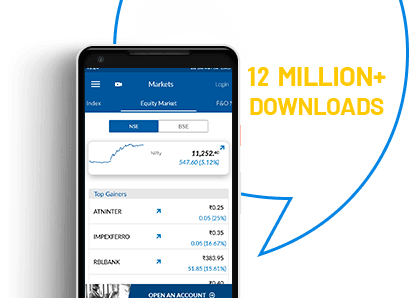# RECURRING DEPOSIT CALCULATOR

Recurring Deposit is a simple term deposit investment tool where you make fixed monthly investments towards it. It is a good option for investors who don’t want to make a lump sum investment in FD, but at the same time wants to grow a regular investment habit. Since it is one of the traditional investment tools, most banks and financial institutions offer Recurring Deposit to their customers.

Although the concept of RD is very straight forward, it involves complex calculation. Earlier, when the process was manual, calculating RD return was cumbersome and had a higher chance of error. But with a modern-day online RD calculator, anyone can easily calculate how much an RD investment will fetch within few seconds.

Angel One RD calculator is simple and user friendly, gives you accurate result in few seconds.

Open a DEMAT Account
& Enjoy FREE Equity Delivery for Lifetime## RD Calculator

Monthly Investment
Number of Months
Months
Rate of Interest
%
0.0
Total Value

## A few things to know about Recurring Deposit before you invest

• It is a traditional investment tool, gives you conservative return in a cumulative manner
• Most banks offer interest between 3.5 and 8.5 percent on recurring deposits
• Most banks will charge a 2 percent penalty for early withdrawal
• RD investment is subjected to taxation. Valid from June 1st, 2015, 10 percent TDS is levied if the investment amount is more than Rs. 40,000. But the tax only applies to the interest amount and not on the maturity value
• You can choose RD tenure between 6 months to 10 year

## FAQs - Frequently Asked Questions

A recurring deposit has certain advantages and disadvantages when compared to other investment options.

• One form of traditional investment. Inculcate the habit of systematic investment in the investor
• One can start even with a small amount
• It can be done at any age. Some banks allow even children to have RD account
• Guaranteed income; suitable for senior citizens and risk-averse investors
• Investors can take a loan against it

• RD is losing popularity due to low return
• Not ideal for young investors with investment goals
• In case of any issues, only Rs 100,00 is covered by RBI
• Since a small amount is invested, earning is also less compared to other investment instruments
• ### Recurring Deposit or stocks, where should I invest?

The ultimate investment decision rests on you. It should depend on your investment goals and abilities to take risks. Here is a comparison between RD and stock investment.
 Areas of concern Recurring Deposit Stocks/Equity Investment Type Monthly fixed investment for over a period No investment commitment. You can invest as little or as much as you want Tenure Fixed tenure - 12 months or 36 months or more No fixed tenure. You can invest for few hours or few days Risk Conservative investment with no risk. Confirmed return at the end of the tenure Subject to market risks Return Compounded interest earning. Low to moderate return Best performing asset class. Historically proven to  offer a better return than any other investment Liquidity Fixed tenure. Early withdrawal may attract penalty Highly liquid Tax Interest over Rs 10,000 is subject to TDS Tax-free return Inflation sensitivity Low Can beat inflation

### What does an RD calculator do?

An online RD interest calculator is a financial tool that helps you calculate maturity value on your recurring deposit quickly and straightforwardly. It helps you get clarity over your investment decision and let you compare between the best RD options available.

In recurring deposit, interest is compounded quarterly using a complex formula. RD calculator takes the hassle out from the process, makes it easy for anybody to calculate the return. It helps investors to make an informed investment decision and align their financial goals.

### What is the formula to calculate RD interest?

RD is calculated using a complex formula. It consists of three variables.

The RD calculator
M = R [(1+i) n – 1]/ 1- (1+i) -1/3
Where,
M = Maturity Amount
R = Recurring Deposit Monthly Instalment
n= Tenure
i= Rate of Interest

Example: Let’s say an investor starts an RD with Rs 500 for 5 years. Payable interested on the deposit is 12 percent. Final maturity amount will be calculated as,
M= 500[(1+12)5-1]/1-(1+12) – 1/3
M= 41, 243

Calculating RD return manually using this formula is difficult and time-consuming. But with RD calculator, you can get the result in seconds.

### How does Angel One RD calculator work?

The RD deposit calculator in Angel One’s website is easy to use and don’t need any technical skills. Here is a guide to use the calculator.
• Enter the monthly amount you’ll deposit
• Enter tenure and expected rate of return
• Click on ‘Calculate’
• The final result will be calculated automatically

• Want to compare different RD investments to find the best one? You can do it easily with RD deposit calculator which calculates results within few seconds.

### What are the advantages of using RD deposit calculator?

There are quite a few.

Easy to use: It can be used by any age group with any level of technical expertise. Keeping in mind that our customer base is diverse, the calculator is designed to accept simple inputs to calculate the outcome. All you have to do is enter the deposit value, tenure, and interest amount; the calculator will compute the maturity amount accurately.

Convenience: The purpose of using an online RD calculator is to save time, and it does the same. It accurately calculates complex formulas within seconds and saves time if you want to compare between different recurring deposit options.

Error-free: The online RD calculator gives error-free results in seconds. It runs on a smart algorithm and returns accurate results if all inputs are given correctly.

Helps financial planning: It enables you to plan your future by calculating possible outcomes with precision. It helps you learn how much you need to invest to achieve your financial goals in advance.

Free of cost: We don’t charge you to use the calculator. You can use it multiple times to compare between different RD outcomes until you find the best solution.

### How is recurring deposit interest calculated?

Expected return on RD depends on its interest income. Using the RD interest calculator, you can avoid the complex manual calculation to compute interest earning on your RD investment.

In recurring deposit, the interest is compounded quarterly. It varies between the banks. But it is also influenced by a few external factors.

RBI policy: RBI decides the repo rate. Repo rate is the interest that banks are required to pay to RBI to borrow fund. If RBI lowers repo rate, it reduces the cost of borrowing for the banks which they pass on to their customers as higher returns.

Recession: Recession slows down economic performance in all sectors, including banks. In case of recession, banks often lower return on RD due to low credit demand.

Inflation: RD interest rate matches the inflation rate in the economy. Higher the inflation rate, higher is the return on RD.

Age of investor: To offer a benefit to senior citizens, banks offer a higher interest rate to them. It will also affect the maturity amount of your RD.

Tenure: RD maturity calculator takes tenure as a variable input to calculate final maturity value since the duration of investment directly impacts the amount of interest earned.

Taxes: RD interest income is subject to TDS deduction. The amount of TDS depends on the annual income of the investor. Banks impose 10 percent TDS on RD interest income if it exceeds Rs 10,000. But only the interest is subject to tax, not the principal amount.

Enjoy Zero Brokerage on Stock Investments